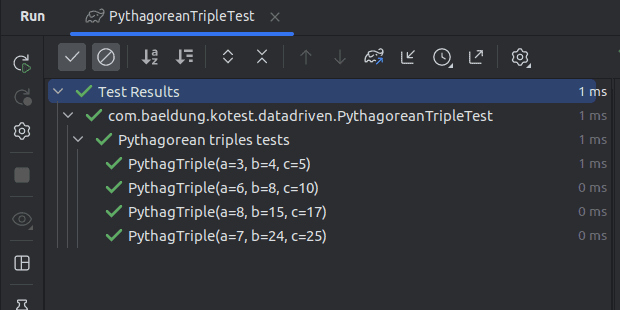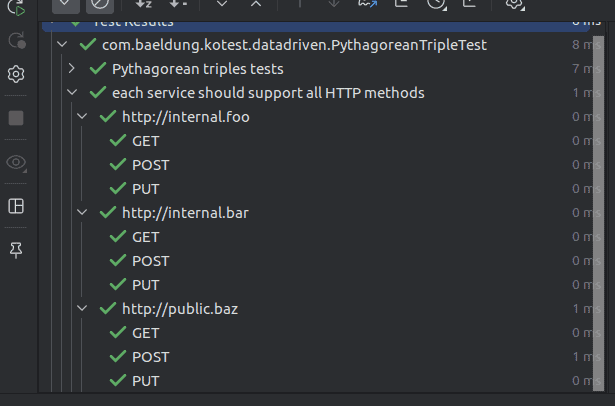## 1. Introduction

Data-driven testing, also known as table-driven testing, is a powerful technique used to validate various combinations of input data against a given function. This approach is particularly useful for testing scenarios where multiple sets of input data need to be tested to ensure that the code behaves as expected across various cases.

Kotest is a flexible and comprehensive testing framework for Kotlin that provides first-class support for data-driven testing, making it easy and efficient to write tests that cover a wide range of input combinations.

In this article, we’ll explore how to leverage Kotest’s data-driven testing capabilities to create robust and comprehensive tests. We’ll walk through basic examples, demonstrate nested data tests, discuss customizing test names, and highlight the benefits of using data-driven testing in our test suite.

## 2. Basic Example: Pythagorean Triangle

Let’s start by considering a common mathematical problem: determining whether a set of three integers forms a Pythagorean triple. A Pythagorean triple consists of three positive integers (a, b, c) that satisfy the equation: a^2 + b^2 = c^2. We want to create tests that verify whether a given set of integers forms a valid Pythagorean triple. Let’s start by writing a function that can find Pythagorean triples – isPythagTriple():

``````fun isPythagTriple(a: Int, b: Int, c: Int): Boolean {
if(a <= 0 || b <= 0 || c <= 0) return false
return a * a + b * b == c * c
}
``````

To test this using data-driven testing, we’ll define a data class to represent a single row of test data. This class will be close to our test, and not the production code:

``data class PythagTriple(val a: Int, val b: Int, val c: Int)``

We can then use instances of this data class to perform data-driven tests. Here’s an example of how we can use data-driven testing with Kotest to test our isPythagTriple() function:

``````class PythagoreanTripleTest : FunSpec({
context("Pythagorean triples tests") {
withData(
PythagTriple(3, 4, 5),
PythagTriple(6, 8, 10),
PythagTriple(8, 15, 17),
PythagTriple(7, 24, 25)
) { (a, b, c) ->
isPythagTriple(a, b, c) shouldBe true
}
}
})
``````In this example, withData() generates multiple test cases based on the provided data rows. The test will be executed for each row, and the expected outcome is specified using the matcher function shouldBe(). If any of the test cases fail, Kotest will provide detailed information about the failing input values.

## 3. Nested Data Tests

One of the strengths of Kotest’s data-driven testing is its support for nested data tests. This allows us to test combinations of input values across multiple dimensions. For example, let’s write a test on how different services respond to various HTTP methods:

``````context("each service should support all HTTP methods") {
val services = listOf(
"http://internal.foo",
"http://internal.bar",
"http://public.baz"
)

val methods = listOf("GET", "POST", "PUT")

withData(services) { service ->
withData(methods) { method ->
// test service against method
}
}
}
``````In this example, Kotest generates a Cartesian product of the input values from both services and methods. This results in comprehensive testing across all our combinations of services and methods.

## 4. Data Tests Naming

By default, Kotest generates test names based on the toString() representation of the input data class. However, we can customize test names to provide more meaningful information.

### 4.1. Stable Names

To ensure stability in test names, Kotest will only use the toString() of the input class if it thinks the input class has a stable toString() value, otherwise, it will use the class name. We can force the use of toString() by annotating our data class with @IsStableType:

``````@IsStableType
data class PythagTriple(val a: Int, val b: Int, val c: Int)``````

### 4.2. Using a Map

We can specify test names by providing a map to the withData() function, where the keys represent test names and the values are the corresponding input for each test:

``````context("Pythagorean triples tests with map") {
withData(
mapOf(
"3, 4, 5" to PythagTriple(3, 4, 5),
"6, 8, 10" to PythagTriple(6, 8, 10),
"8, 15, 17" to PythagTriple(8, 15, 17),
"7, 24, 25" to PythagTriple(7, 24, 25)
)
) { (a, b, c) ->
isPythagTriple(a, b, c) shouldBe true
}
}``````

### 4.3. Test Name Function

We can also pass a function to the withData() function to dynamically generate test names based on the input data:

``````context("Pythagorean triples tests with name function") {
withData(
nameFn = { "\${it.a}__\${it.b}__\${it.c}" },
PythagTriple(3, 4, 5),
PythagTriple(6, 8, 10),
PythagTriple(8, 15, 17),
PythagTriple(7, 24, 25)
) { (a, b, c) ->
isPythagTriple(a, b, c) shouldBe true
}
}
``````

### 4.4. WithDataTestName Interface

Another alternative for customizing test names is to implement the WithDataTestName interface. Kotest invokes the dataTestName() function for each data class to generate the test name when we implement this interface.

Let’s continue with our Pythagorean triple example and implement the WithDataTestName interface:

``````data class PythagTriple(val a: Int, val b: Int, val c: Int) : WithDataTestName {
override fun dataTestName() = "Pythagorean Triple: \$a, \$b, \$c"
}``````

## 5. Conclusion

Data-driven testing is a powerful approach to thoroughly test our code across various input scenarios. Kotest’s built-in support for data-driven testing simplifies the process of creating tests for different combinations of input data, allowing us to identify and fix issues more effectively.

In this article, we’ve explored the basics of data-driven testing with Kotest, including creating tests using data classes, nesting data tests, and customizing test names. By harnessing the capabilities of data-driven testing, you can enhance the quality and reliability of your Kotlin applications while reducing the effort required to write comprehensive test suites. As always, the code used in this article is available over on GitHub.

Subscribe
Notify of